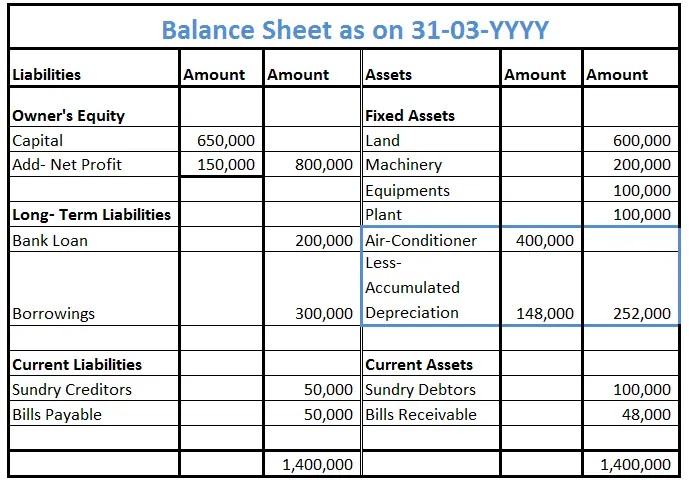# Can assets have a credit balance?

-This question was submitted by a user and answered by a volunteer of our choice.

Yes, there are a few assets that show the credit balance. Those assets generally hold zero or unfavourable balances.

## Assets that have a credit balance

From accounting perspective assets and expenses generally have a debit balance whereas liabilities, revenue and capital have a credit balance. Yet there exist a couple of assets that do have a credit balance those assets are known as contra assets.

## Contra Asset

A contra asset is referred to as an asset that generally has a zero or negative balance. Such an asset is used to offset or reduce the balance of the respective asset account with which it is paired to. Hence reducing or offsetting the amount of the respective asset account with the contra asset account gives us the net value of the respective asset.

It acts as an asset holding credit balance. Contra assets are useful for the organization because it allows them to follow the matching principle by initially recording an expense in the contra asset account.

## Assets with a negative balance

For Example – Max purchased an air conditioner from eBay for 4,00,000. The salvage value of the air- conditioner is 30,000 and has an expected useful life of 10 years. On 31-12-YYYY, how much balance will be shown in the Accumulated Depreciation account.

Calculation

Annual Depreciation = (Value of Asset – Salvage value)/Estimated life of the asset.

= (4,00,000 – 30,000)/10  => 37,000

Dr                                       Accumulated Depreciation a/c                                     Cr

 Date Particulars Amount Date Particulars Amount 31-12-YYYY By Dep. a/c 37,000 31-12-YYYY By Dep. a/c 37,000 31-12-YYYY By Dep. a/c 37,000 31-12-YYYY By Dep. a/c 37,000 Total 1,48,000

Net Asset value = Total asset value – Accumulated Depreciation

= 4,00,000 – 1,48,000  => 2,52,000

## Placement in the Balance SheetHere in the balance sheet “Accumulated Depreciation” shows a negative balance which is a contra asset and it is deducted from the respective asset account. Hence providing us with the Net value of the asset.

###### Subscribe
* indicates required